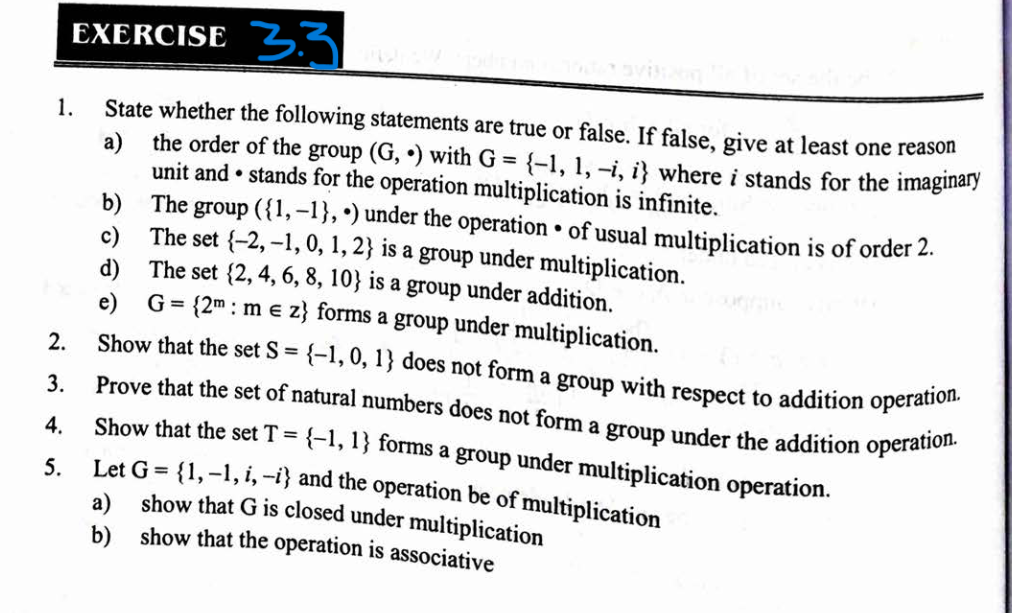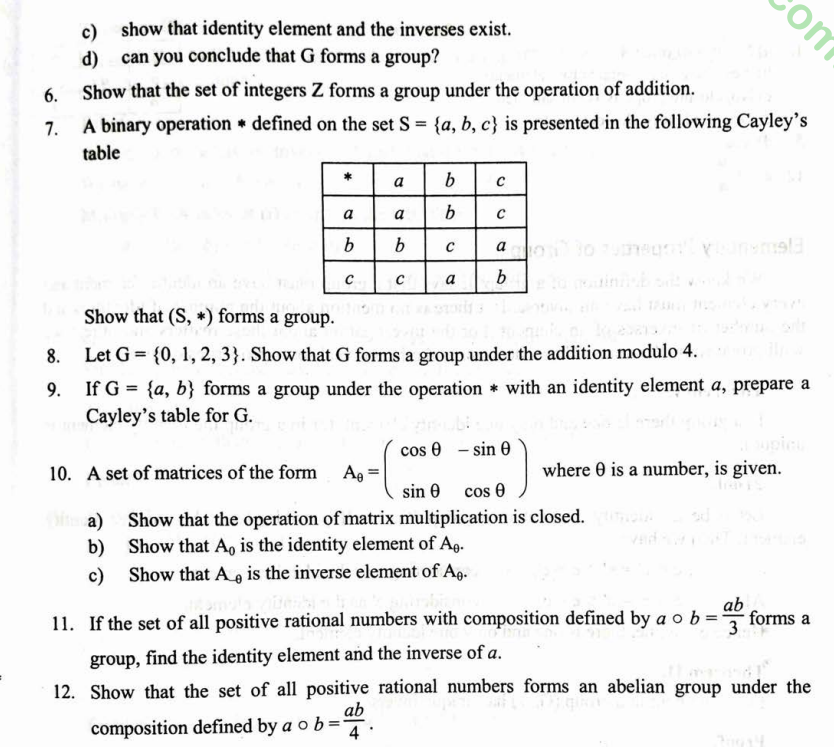# Elementary Group Theory - Exercise 3.3 : Class 12 Math

Complete Exercise 3.3 Elementary Group Theory. Exercise 3.2: Groups Complete Que Ans note, Class 12 Mathematics.

## Chapter 1: Elementary Group Theory.

### Exercise: 3.3

Complete Exercise of Elementary Group Theory - Exercise 3.3 : Class 12 Mathematics 2080 NEB.

Exercise 3.2 is about: Group and its types: Finite and infinite group, Trivial group, Abelian group Groups with elements other than numbers, Matrix Group are also include in this chapter.

Elementary Group Theory Exercise 3.3 Questions#### Question no. 1

a.

Soln:

It is a false statement because the order of group G = Number elements in G = 4, which is finite.

b.

It is a true statement because the group contains two elements, so the order of given group is 2.

c.

Soln:

If is a false statement because it is not closed, for e.g.

(- 2).2 = - 4 ∉ {-2,-1,0,1,2}

d.

Soln:

It is false statement because it is not closed,

For e.g. 4 + 8 = 12 ∉ {2,4,6,8,10}

#### Question no. 2

Soln:

Here, 1 + 1 = 2 ∉ S where, 1 , 1 ԑ S.

So, S is not closed under addition.

Thus, S is not a group under addition.

#### Question no. 3

Soln:

Existence of Identity: Let a be any natural number and if it exists, let e be the identity element of a, then a + e = a. à e = a – a = 0 which is not a natural number,

So, identity element under addition doesn’t exist in the set of natural numbers,

Hence, the set of natural numbers does not form a group under the addition operation,

#### Question no. 4

Soln:

Closure property: From the multiplication table, we see that T is closed under multiplication, i.e.

Multiplication Table.

 X -1 1 -1 1 -1 1 -1 1

(-1) * (-1) = 1, (-1) * 1 = -1

1 * (-1) = - 1, 1 * 1 = 1

So, a * b ԑ T for all a,b ԑ T.

Associative property: Here, the elements of T are – 1 and 1. We know that all integers under multiplication obey associative law. So, the element of T being integers satisfy associative law under multiplication.

i.e. a * b(b * c) = (a * b) * c for all a,b,c, ԑ T.

For e.g. (-1) * {1 * (-1)} = (-1) * (-1) = 1.

And {(-1) * 1} * (-1) = (-1) * (-1) = 1

So, (-1) * {1 * (-1} = {(-1) * 1} * (-1) and so on.

Existence of Identity element,

Since (-1) * 1 = (-1) = 1 * (-1)

And 1 * 1 = 1

So, 1 is the multiplicative identity in T.

Existence of inverse

Each element of T is an inverse of itself since,

1 * 1 = 1 and (-1) * (-1) = 1.

Hence, T = {-1,1} forms a group under multiplication.

#### Question no. 5

a.

Soln:

Multiplication Table.

 X 1 -1 i -i 1 1 -1 i -i -1 -1 1 -i i i i -i -1 1 -i -i i 1 -1

From above table, we see that for al a,b ԑ G, a * b ԑ G. So G is closed under multiplication.

b.

Soln:

Since all the complex numbers satisfy associative law under multiplication. So all the elements of G being complex numbers also satisfy associative law.

Ie. a * (b * c) = (a * b) * c, for all a,b,c ԑ G.

c.

Soln:

For all a ԑ G, a * 1 = 1 * a = a.

So, 1 is the multiplication identity in G.

Since, 1 * 1 = 1

(-1) * (-1) = 1

i * (-i) = -i2 = 1

and (-i) * I = -i2 = 1

So, the inverse of 1, -1, i and – I and 1, - 1, - i and i , respectively. So ,every element of G possesses an inverse element in G. Hence, identity element and inverse exists.

d.

Soln:

Yes, G forms a group under multiplication as (G,x) is closed, associative, and the identity and inverse exist in G.

#### Question no. 6

Soln:

Let a,b ԑ Z.

Since, a,b ԑ Z à a + b ԑ Z.

So, closure property is satisfied.

If a,b,c ԑ Z, then

a + (b + c) = a + b + c ԑ Z.

Also, (a + b) + c = a + b + c ԑ Z.

So, a + (b + c) = (a + b) + c.

Hence, associative property is satisfied. 0 is an integer and

0 + a = a + 0 = a ԑ Z.

0 is an identity element.

Also, if a ԑ Z then – a ԑ Z.

Or, a + (- a) = (-a) + a = 0.

So, - a is the inverse element of a. Above relations are true for all elements of Z.

Hence, the set of integers Z forms a group under addition.

#### Question no. 7

Soln:

 * a b c a a b c b b c a c c a b

From the table, we see that the operation defined on any two elements of G gives and element of G itself.

So, G is closed under the operation *.

a * (b * c) = a * a = a

(a * b) * c = b * c = a

So, a * (b * c) = (a *b) * c

So, * satisfies associative property.

Since, a * a = a, a * b = b * a= b

And a * c = c * a = c, so a is an identity element.

a * a = a, so a is the inverse of a,b * c = c * b = a, so b and c are the inverse elements of c and  respectively,

So, (S,*) forms a group.

#### Question no. 8

Soln:

Composition table for G under the addition modulo r (+4) is presented below.

 +4 0 1 2 3 0 0 1 2 3 1 1 2 3 0 2 2 3 0 1 3 3 0 1 2

From the table, we see that sum of any two elements of G modulo 4 is an element of G. So, +4 satisfies closure property.

Again, 1 +4 (2 +4 3) = 1 +4 1 = 2.

And (1 +4 2) +4 3 = 3 +4 3 = 2.

This result is true for all elements of G. Hence, +4 satisfies associative property.

From the second row and second column of above table, 0 is the identity element.

Form the second row and second column of above table, 0 is the identity elemnt.

Since, 0 +4 0 = 0, 1 +4 3 = 3 +4 1 = 0

And 2 +4 2 = 0

So, the inverse elements of 0,1,2 and 3 are 0,3,2 and 1 respectively.

So, G forms a group under addition modulo 4.

#### Question no. 9

Soln:

Since a is an identity element, so,

a * a = a,  a * b = b * a = a.

So, also a * a = a, a is the inverse of a.

And b * b = a so that b is the inverse of b.

Now the required composite table is given below.

 * a b a a b b b a

### Elementary Group Theory - Exercise 3.3 : Class 12 Math PDF

Elementary Group Theory - Exercise 3.1 : Class 12 Math

Elementary Group Theory - Exercise 3.2 : Class 12 Math

Elementary Group Theory - Exercise 3.4 : Class 12 Math

Getting Info...